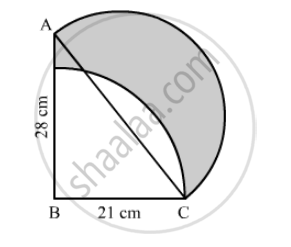Advertisement Remove all ads

# In the Following Figure, Abc is a Right-angled Triangle, ∠B = 90°, Ab = 28 Cm and Bc = 21 Cm. with Ac as Diameter a Semicircle is Drawn and with Bc as Radius a Quarter Circle is D - Mathematics

Sum

In the following figure, ABC is a right-angled triangle, ∠B = 90°, AB = 28 cm and BC = 21 cm. With AC as diameter a semicircle is drawn and with BC as radius a quarter circle is drawn. Find the area of the shaded region correct to two decimal places.Advertisement Remove all ads

#### Solution

We have given two semi-circles and one circle.

Area of the shaded region = area of semicircle with diameter AC + area of right angled triangle ABC − area of sector

First we will find the hypotenuse of right angled triangle ABC.

AC^2=AB^2+BC^2

∴ AC^2=28^2+21^2

∴ AC^2=784+441

∴ AC^2=1225

∴ AC=35

∴ "Area of the shaded region"=(pixx17.5xx17.5)/2+1/2xx28xx21-θ/360xxpixx21xx21

∴ "Area of the shaded region"=(pixx17.5xx17.5)/2+14xx21-1/4xxpixx21xx21

Substituting pi=22/7we get,

∴ "Area of shabed region"=(22/7xx17.5xx17.5)/2+14xx21-1/4xx22/7xx21xx21

∴ "Area of shabed region"=962.5/2+14xx21-1/2xx11xx3xx21

∴ "Area of shabed region"=481.25+294-346.5

∴ "Area of shabed region"=428-75

Therefore, area of shaded region is 428.75 cm^2

Is there an error in this question or solution?
Advertisement Remove all ads

#### APPEARS IN

RD Sharma Class 10 Maths
Chapter 13 Areas Related to Circles
Exercise 13.4 | Q 40 | Page 62
Advertisement Remove all ads

#### Video TutorialsVIEW ALL 

Advertisement Remove all ads
Share
Notifications

View all notifications

Forgot password?
Course